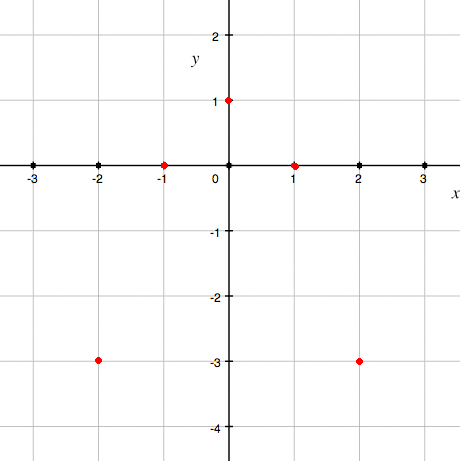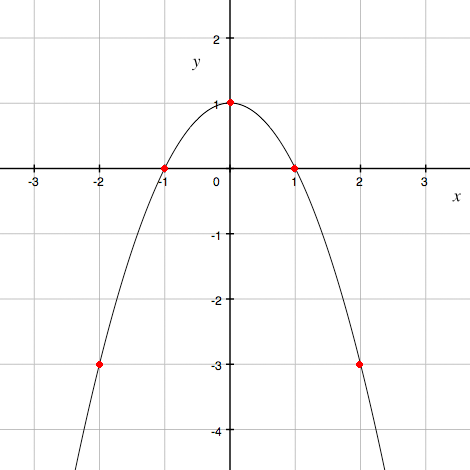SEARCH HOMEMath Central Quandaries & QueriesQuestion from Max, a student: How do I graph y = -2x^2?Hi Max,

I would start by plotting some points. Let me show you with y = -x2 + 1. I chose values x = 0, 1, -1, 2 and -2 and for each I found the value of y using y = -x2 + 1.

x y
0 1
1 0
-1 0
2 -3
-2 -3

Then I plotted these points on an xy coordinate systemThen I sketched a graph through these points